# DAV Class 5 Maths Chapter 6 Worksheet 2 Solutions

The DAV Maths Book Class 5 Solutions and DAV Class 5 Maths Chapter 6 Worksheet 2 Solutions of Addition and Subtraction of Decimal Numbers offer comprehensive answers to textbook questions.

## DAV Class 5 Maths Ch 6 Worksheet 2 Solutions

Question 1.
Complete the following:
(a)Solution:(b)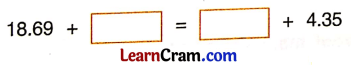Solution: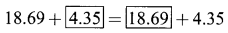(c)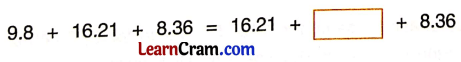Solution: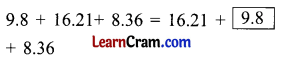(d)Solution: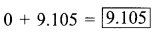(e)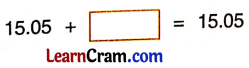Solution: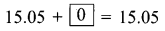(f)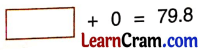Solution:(g)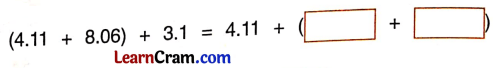Solution: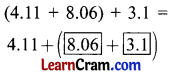(h)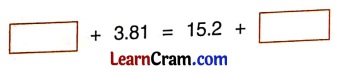Solution:DAV Class 5 Maths Chapter 6 Worksheet 2 Notes

If we change the order of addends the sum remains the same.

Example 1.
Solution:
3.6 + 5.2 = 8.8
5.2 + 3.6 = 8.8
The sum remains the same, even after changing the groupings of addends.

Example 2.